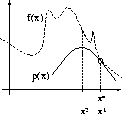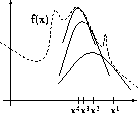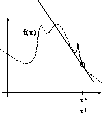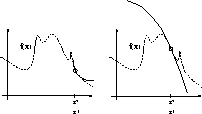Next: Placing and Tightening the Up: Optimization - General Bound Previous: Quadratic Bounds

# Maximizing with Bounds: Beyond Gradient Ascent

Now consider the use of a bound such as the one proposed above. We have an analytically specified function, f, which we are trying to optimize starting from an initial point x* = x1. Figure 6.1 depicts the bound and the function to be optimized. Note the contact point where the function meets the bound is at x*.We can now find the maximum of that bound and move the contact point x* to this new locus on the function. This point is now x2 and is used to find another parabola which lies below the function f and touches it at our current operating point, x2. As this process is iterated, we gradually converge to a maximum of the function. This process is illustrated in Figure 6.2. At the function's maximum, however, the only parabola we can draw which is a lower bound is one whose peak is at the function's maximum (i.e. a 'fixed' point). The algorithm basically stops there. Thus, there is a natural notion of a fixed point approach. Such an algorithm will always converge to a local maximum and stay there once locked on.In the example, the algorithm skipped out of a local maximum on its way to its destination. This is not due to the fact that the local maximum is lower but due to the some peculiarity of the bounding approach and its robustness to very local attractor. The bound is shaped by the overall structure function which has a wider and higher local maximum at xfinal. We will discuss this trade-off between the amplitude of a local maximum and its robustness (width of the basin) later on and their relationship to annealing and global optimization.However, gradient and Taylor approximation approaches have remained popular because of their remarkable ease of use and general applicability. Bound techniques (such as the Expectation Maximization or EM algorithm ) have not been as widely applicable. This is because almost any analytic function can be differentiated to obtain a gradient or higher order approximation. However, we shall show some important properties of bounds which should illustrate their wide applicability and usefulness. In addition, some examples of nonlinear optimization and conditional density estimations will be given as demonstrations.Next: Placing and Tightening the Up: Optimization - General Bound Previous: Quadratic Bounds
Tony Jebara
1999-09-15